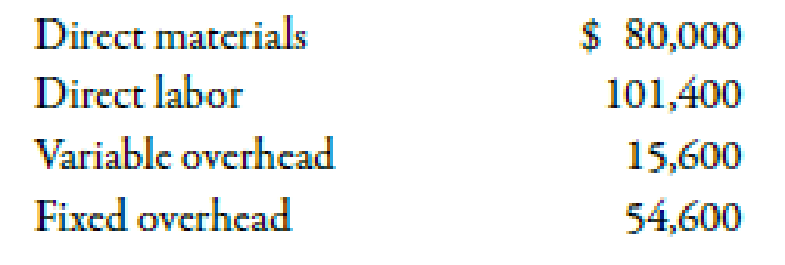Chapter 3, Problem 52E### Managerial Accounting: The Corners...

7th Edition
Maryanne M. Mowen + 2 others
ISBN: 9781337115773

#### Solutions

Chapter
Section### Managerial Accounting: The Corners...

7th Edition
Maryanne M. Mowen + 2 others
ISBN: 9781337115773
Textbook Problem
1 views

# Inventory Valuation under Absorption CostingAmiens Company produced 20,000 units during its first year of operations and sold 18,900 at \$17 per unit. The company chose practical activity—at 20,000 units—to compute its predetermined overhead rate. Manufacturing costs are as follows:Required: 1. Calculate the unit cost for each of these four costs. 2. Calculate the cost of one unit of product under absorption costing. 3. How many units are in ending inventory? 4. Calculate the cost of ending inventory under absorption costing.

1.

To determine

Compute the value of unit costs for each of the given cost.

Explanation

Cost:

Cost can be defined as the cash and cash equivalent which is incurred against the products or its related services which will benefit the organization in the future. There are two types of costs that are fixed and variable costs.

Use the following formula to calculate unit costs:

Unit Cost=Manufacturing CostNumber Of Units

 Unit Cost st

2.

To determine

Compute the value of per unit cost of product with the help of absorption costing.

3.

To determine

Calculate the ending inventory units.

4.

To determine

Compute the value of ending inventory with the help of absorption costing.

### Still sussing out bartleby?

Check out a sample textbook solution.

See a sample solution

#### The Solution to Your Study Problems

Bartleby provides explanations to thousands of textbook problems written by our experts, many with advanced degrees!

Get Started